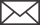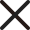# Analysis of fabric weave

2022-04-23   Pageview：166

As mentioned above, the weave of the fabric is the law that the warp and weft are interlaced with each other, and the knitted fabric is the law that the threads and ⭕ are intertwined with each other. When the density of the fabric is low and the structure is relatively simple, the structure can be drawn directly as long as it is observed with an analytical mirror (illumination). When the organization is more complex or the density is large, the fabric organization needs to be analyzed by the pull-out method.

The so-called plucking method is to observe the interweaving law of warp and weft yarns under the plucked state of the fabric using an analytical mirror. The specific method is to first remove the warp and weft of the sample by about 1cm along the edge, leaving the yarn tassel, and then under the analysis mirror, use a needle to poke the first warp yarn (or weft yarn) away, so that there is a slight gap between it and the second yarn , and place it in the middle of the weft yarns, so as to observe the interlacing law of the warp yarns and the weft yarns, and make a record (draw it directly on the graph paper or artisan paper). This warp yarn is then removed and the second warp yarn is observed in the same way until the number of warp cycles is reached.When analyzing, it should be noted that the first analysis is the warp, then the warp is still analyzed below, and the warp and weft cannot be exchanged for analysis; the second point to pay attention to is that once the analysis starts, in addition to the recorded yarn can be removed, other The yarns of a system and the unanalyzed yarns of the same system cannot be drawn out randomly, textile sizing wax manufacturers otherwise the analysis must be restarted.

If a piled fabric is encountered, the fluff of the fabric should be sheared or burned before the analysis, and then the analysis is carried out. When re-tissue or double-tissue analysis, it must be done layer by layer.

Analysis of warp and weft yarn thickness

The analysis of the thickness of warp and weft yarns is relatively simple, as long as the yarns in the fabric are extracted, weighed, and calculated according to the linear density calculation formula after dyeing

At present, the international commonly used system is Tex, the short fiber is represented by tex, and the long fiber is represented by dtex.

Linear Density of Yarn and Its Representation

Yarn is a thin and long object, the length is many times larger than the diameter, the thickness of the yarn is called the linear density,

Therefore, linear density is an indicator that describes the thickness of yarns and threads.

The linear density can be directly expressed by the cross-sectional area or diameter, but the yarn is easily deformed and has an irregular cross-section, so it is often expressed by indirect indicators. The commonly used indirect linear density indicators are:

Linear Density of Staple Yarn

Before 1987, the textile industry was accustomed to expressing the linear density of cotton yarn with the inch count Ne; used to express the thickness of wool yarn, hemp yarn and silk thread with the metric count Nm. The formula for calculating the inch count is as follows:

Ne=Le÷840GeLe and Ge are the British length yards and the British official weight pounds, respectively.

The conversion formula of imperial count and tex is as follows:

Nt=590.54÷Imperial count × [(100+Tex metric moisture regain (%))÷(100+Imperial metric moisture regain (%))]=conversion factor÷Ne

The conversion factor varies with the inch moisture regain, and the conversion factor of pure cotton yarn is 583.1.

The calculation formula for the metric count is: Nm = yarn length ÷ yarn weight

The linear density index of yarns spun from short fibers is uniformly expressed in tex, and the unit is tex, which is abbreviated as tex in Chinese. The physical meaning of tex is the number of grams of a given weight of yarn with a length of 1000m. So the tex calculation formula is as follows:

tex = the official weight of the yarn (g) ÷ the length of the yarn (m) × 1000

The conversion formula of metric count and tex is:

tex=1000÷Nm

Commonly used cotton yarn specifications are 13tex14.5tex19.4tex, 29tex, 32tex, etc., commonly used wool yarn specifications are 11.8tex, 9.8tex, 12.3tex, 15.5tex, etc.; commonly used silk specifications are 5.9tex, 4.9tex, etc.

Linear Density of Filament – The linear density of chemical filaments used to be expressed in denier or metric count.

The linear density of natural silk and chemical thread filaments is commonly expressed as fineness. The so-called fineness refers to the number of grams of the official weight of the silk thread or fiber per unit length. It used to be expressed in denier. Uniform use of tex or dtex.

Commonly used natural silk size specifications are: mulberry silk 22/24.2dex, 14.3/16.5dex, 30.8/33dtex; tussah silk 38.5dtex, 77dtex. The common specifications of chemical filaments are: 66dex, 82.5dex, 132dex for viscose; 82.5dex, 165dex for polyester filament, etc.

Representation of yarn specifications – denier refers to the number of grams of the official weight of 9000m long filament, the conversion formula of denier and tex is: 1 denier = 0.11tex

At present, the expression of linear density and twist has not been unified into the national standard number system for work, denier system, and imperial count, which is very non-standard, such as 2/20/22 – means two 20~22 denier Composite silk made of Neil silk. (2/20/22×18T+20/22)16T/Z——indicates that a 2-strand 20~22 denier twisted yarn with 18 twists/cmS twist is combined with a 20~22 denier untwisted yarn Add a twist of 16 twists/cmZ twist. 120D/30F—represents a composite chemical fiber with a linear density of 120 denier synthesized from 30 monofilaments.

60/3——represents a twisted yarn made of three 60-inch yarns twisted together. No. 7.5 × 3—represents a twisted yarn formed by twisting three single yarns of No. 7.5 in parallel. These representation methods obviously do not conform to the unified national standards, but they are often used in production and transactions. Therefore, it is an urgent problem to unify the representation method of line density into the national standard as soon as possible.

The specifications of the yarn should indicate the thickness of the yarn, whether it is twisted, the twist and twist direction, and the number of strands combined. For the representation of yarn fineness, twist, twist direction and the number of combined strands, national standards all stipulate, and the tex system is uniformly used.

The single yarn is expressed as: special number tex (twist direction) (twist)

Such as 40texZ660, it means 40tex single yarn, twist direction Z, twist is 660 twists/m. For untwisted filament, it is expressed as:

(Special number) tex (single yarn twist direction) (single yarn twist) × (the number of plies) (ply twist direction) (ply twist)

For example, 34texS600×2Z400 means: 34tex, its twist direction is S twist, 600 twists/m, 2 strands are combined, and the combined twist direction is Z twist, and the twist is 400 twists/m. For untwisted filament, it is expressed as: (dtex) dtex f (number of single filaments) to

For example, 133dtexf40to means that the filament of 133dtex is not twisted after merging 40 monofilaments, to is the symbol of no twist, and f is the symbol of filament.

For twisted filaments, it is expressed as: (dtex) dex f (number of single filaments) (twist direction) (filament twist) R (dtex after twisting) dtex

For example, 133dtexf40S1000R136dtex means that the filament with linear density of 133dtex is composed of 40 monofilaments, the twist direction is S twist, the twist is 1000 twists/mm, and the linear density after twisting is 136dtex.

Twist and twist direction analysis of warp and weft yarns

The twist and twist direction of the warp and weft yarns can significantly affect the appearance and feel of the fabric, and the twist is the number of twists per unit length of the yarn. Therefore, to measure the twist, a twist tester can be used, or it can be measured manually. The specific measurement method is to take a certain length of yarn, one end is fixed, the other end is rotated, the direction of rotation is opposite to the direction of twisting, so that it is untwisted, and the number of revolutions after the twist is withdrawn is calculated. number of times. There are two twist directions of yarn twisting: Z twist and S twist. When analyzing the twist direction, as long as you hold both ends of the yarn by hand, if the upper end is fixed and the lower end can be untwisted by rotating clockwise, it is S twist; if the lower end rotates counterclockwise to untwist, it is Z twist.

Yarn is a product of a certain thickness processed from various textile fibers, which is used for weaving, thread making, rope making, knitting and embroidery. Yarns are used for different purposes, but the basic structure is the same. It is usually called yarn that is directly spun from short fibers; the yarn that is combined with two or more yarns is called thread. In recent years, due to the improvement and innovation of new spinning processes, a variety of new types of yarns have emerged with decidedly different structures, properties and appearances.

*
*

• Lastest News
• Rand News
• ## You May LikeLeave a message

Contact UsContact Us